Anzeige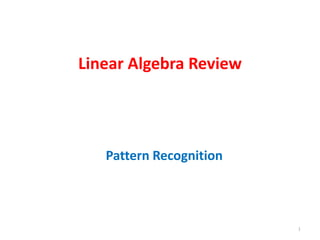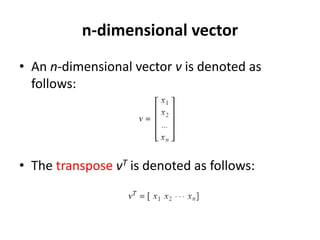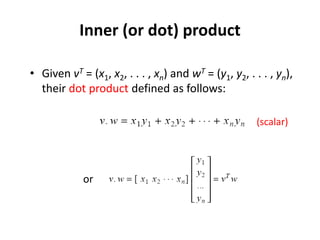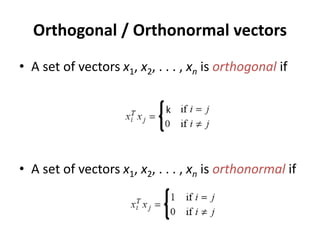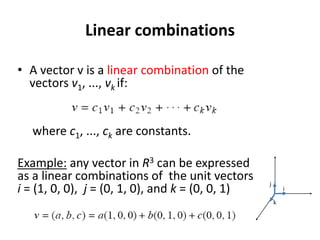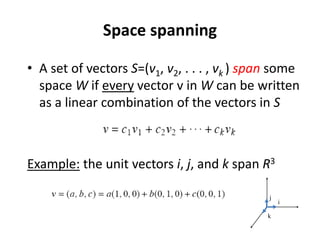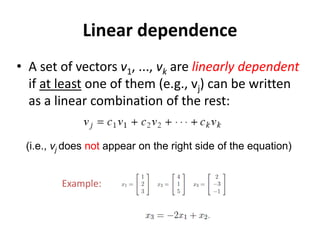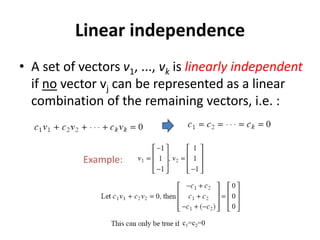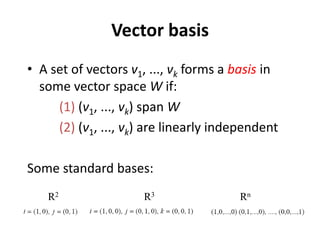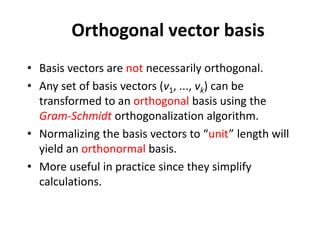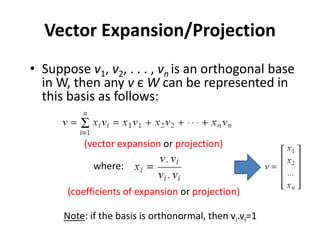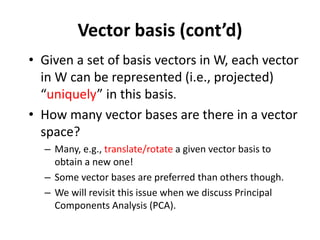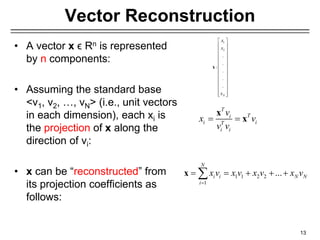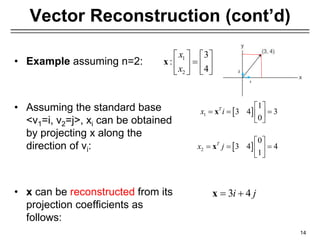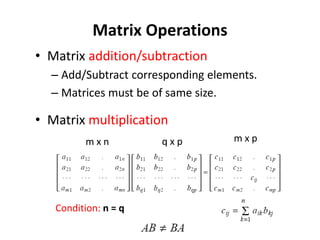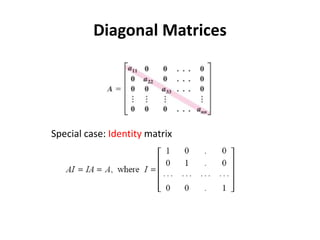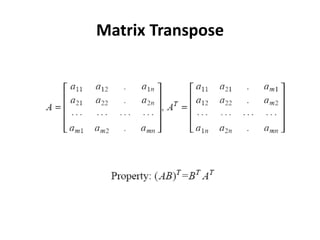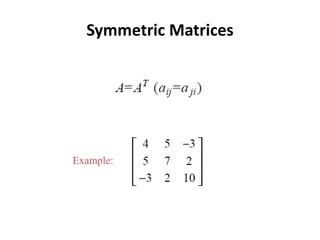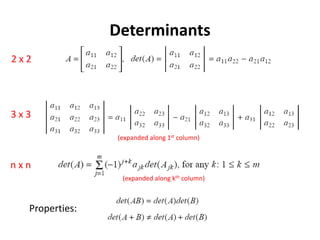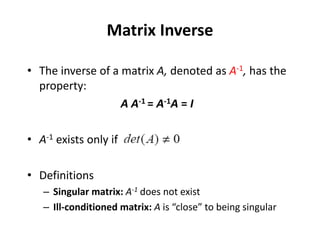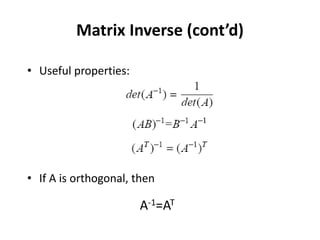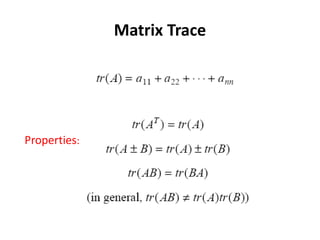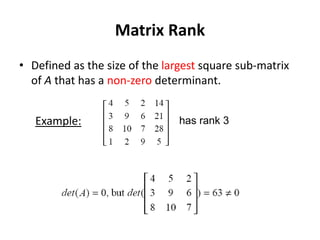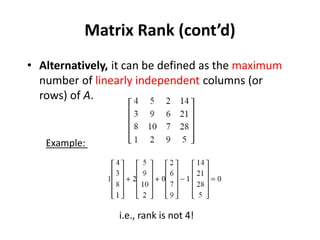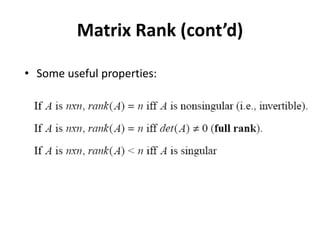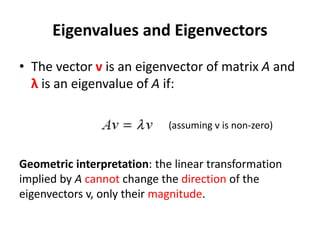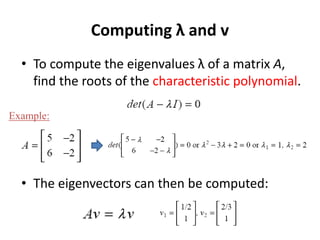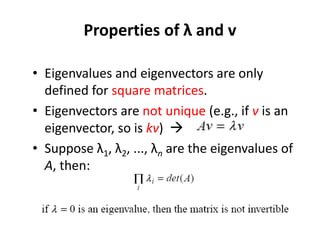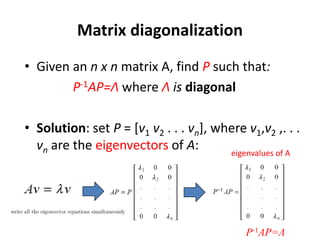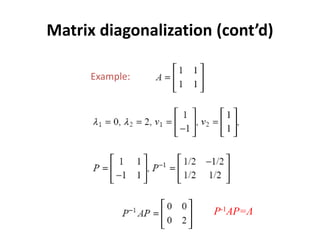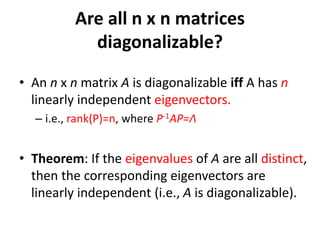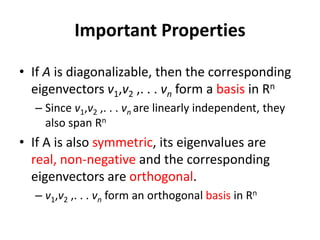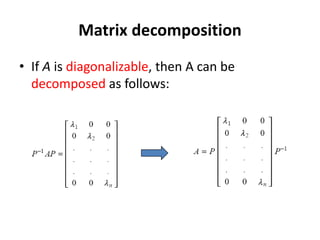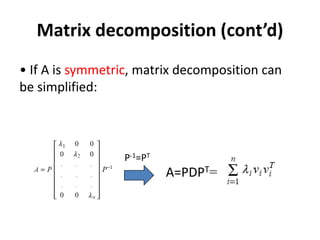1 von 34
Anzeige

### LinearAlgebraReview.ppt

1. Linear Algebra Review 1 Pattern Recognition
2. n-dimensional vector • An n-dimensional vector v is denoted as follows: • The transpose vT is denoted as follows:
3. Inner (or dot) product • Given vT = (x1, x2, . . . , xn) and wT = (y1, y2, . . . , yn), their dot product defined as follows: or (scalar)
4. Orthogonal / Orthonormal vectors • A set of vectors x1, x2, . . . , xn is orthogonal if • A set of vectors x1, x2, . . . , xn is orthonormal if k
5. Linear combinations • A vector v is a linear combination of the vectors v1, ..., vk if: where c1, ..., ck are constants. Example: any vector in R3 can be expressed as a linear combinations of the unit vectors i = (1, 0, 0), j = (0, 1, 0), and k = (0, 0, 1) k j i
6. Space spanning • A set of vectors S=(v1, v2, . . . , vk ) span some space W if every vector v in W can be written as a linear combination of the vectors in S Example: the unit vectors i, j, and k span R3 k j i
7. Linear dependence • A set of vectors v1, ..., vk are linearly dependent if at least one of them (e.g., vj) can be written as a linear combination of the rest: (i.e., vj does not appear on the right side of the equation) Example:
8. Linear independence • A set of vectors v1, ..., vk is linearly independent if no vector vj can be represented as a linear combination of the remaining vectors, i.e. : Example: c1=c2=0
9. Vector basis • A set of vectors v1, ..., vk forms a basis in some vector space W if: (1) (v1, ..., vk) span W (2) (v1, ..., vk) are linearly independent Some standard bases: R2 R3 Rn
10. Orthogonal vector basis • Basis vectors are not necessarily orthogonal. • Any set of basis vectors (v1, ..., vk) can be transformed to an orthogonal basis using the Gram-Schmidt orthogonalization algorithm. • Normalizing the basis vectors to “unit” length will yield an orthonormal basis. • More useful in practice since they simplify calculations.
11. Vector Expansion/Projection • Suppose v1, v2, . . . , vn is an orthogonal base in W, then any v є W can be represented in this basis as follows: • The xi of the expansion can be computed as follows: (vector expansion or projection) (coefficients of expansion or projection) where: Note: if the basis is orthonormal, then vi.vi=1
12. Vector basis (cont’d) • Given a set of basis vectors in W, each vector in W can be represented (i.e., projected) “uniquely” in this basis. • How many vector bases are there in a vector space? – Many, e.g., translate/rotate a given vector basis to obtain a new one! – Some vector bases are preferred than others though. – We will revisit this issue when we discuss Principal Components Analysis (PCA).
13. Vector Reconstruction • A vector x ϵ Rn is represented by n components: • Assuming the standard base <v1, v2, …, vN> (i.e., unit vectors in each dimension), each xi is the projection of x along the direction of vi: • x can be “reconstructed” from its projection coefficients as follows: 13 1 2 . . : . . . N x x x                           x T T i i i T i i v x v v v   x x 1 1 2 2 1 ... N i i N N i x v x v x v x v        x
14. Vector Reconstruction (cont’d) • Example assuming n=2: • Assuming the standard base <v1=i, v2=j>, xi can be obtained by projecting x along the direction of vi: • x can be reconstructed from its projection coefficients as follows: 14 1 2 3 : 4 x x              x   1 1 3 4 3 0 T x i          x 3 4 i j   x   2 0 3 4 4 1 T x j          x i j
15. Matrix Operations • Matrix addition/subtraction – Add/Subtract corresponding elements. – Matrices must be of same size. • Matrix multiplication Condition: n = q m x n q x p m x p n
16. Diagonal Matrices Special case: Identity matrix
17. Matrix Transpose
18. Symmetric Matrices Example:
19. Determinants 2 x 2 3 x 3 n x n Properties: (expanded along 1st column) (expanded along kth column)
20. Matrix Inverse • The inverse of a matrix A, denoted as A-1, has the property: A A-1 = A-1A = I • A-1 exists only if • Definitions – Singular matrix: A-1 does not exist – Ill-conditioned matrix: A is “close” to being singular
21. Matrix Inverse (cont’d) • Useful properties: • If A is orthogonal, then A-1=AT
22. Matrix Trace Properties:
23. Matrix Rank • Defined as the size of the largest square sub-matrix of A that has a non-zero determinant. Example: has rank 3
24. Matrix Rank (cont’d) • Alternatively, it can be defined as the maximum number of linearly independent columns (or rows) of A. i.e., rank is not 4! Example:
25. Matrix Rank (cont’d) • Some useful properties:
26. Eigenvalues and Eigenvectors • The vector v is an eigenvector of matrix A and λ is an eigenvalue of A if: Geometric interpretation: the linear transformation implied by A cannot change the direction of the eigenvectors v, only their magnitude. (assuming v is non-zero)
27. Computing λ and v • To compute the eigenvalues λ of a matrix A, find the roots of the characteristic polynomial. • The eigenvectors can then be computed: Example:
28. Properties of λ and v • Eigenvalues and eigenvectors are only defined for square matrices. • Eigenvectors are not unique (e.g., if v is an eigenvector, so is kv)  • Suppose λ1, λ2, ..., λn are the eigenvalues of A, then:
29. Matrix diagonalization • Given an n x n matrix A, find P such that: P-1AP=Λ where Λ is diagonal • Solution: set P = [v1 v2 . . . vn], where v1,v2 ,. . . vn are the eigenvectors of A: eigenvalues of A P-1AP=Λ
30. Matrix diagonalization (cont’d) Example: P-1AP=Λ
31. • An n x n matrix A is diagonalizable iff A has n linearly independent eigenvectors. – i.e., rank(P)=n, where P-1AP=Λ • Theorem: If the eigenvalues of A are all distinct, then the corresponding eigenvectors are linearly independent (i.e., A is diagonalizable). Are all n x n matrices diagonalizable?
32. • If A is diagonalizable, then the corresponding eigenvectors v1,v2 ,. . . vn form a basis in Rn – Since v1,v2 ,. . . vn are linearly independent, they also span Rn • If A is also symmetric, its eigenvalues are real, non-negative and the corresponding eigenvectors are orthogonal. – v1,v2 ,. . . vn form an orthogonal basis in Rn Important Properties
33. Matrix decomposition • If A is diagonalizable, then A can be decomposed as follows:
34. Matrix decomposition (cont’d) • If A is symmetric, matrix decomposition can be simplified: P-1=PT A=PDPT=
Anzeige